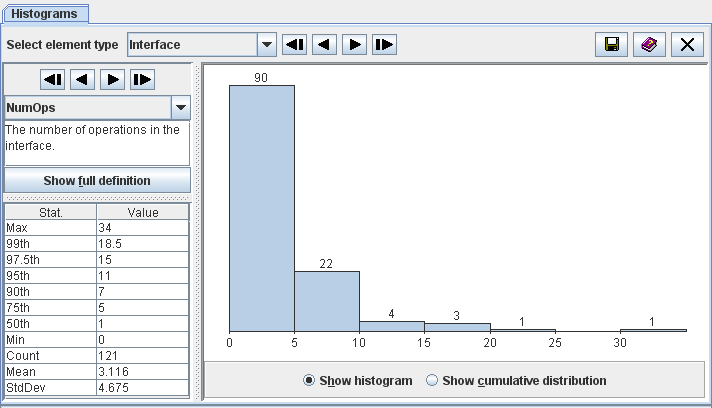Contents > 4 The SDMetrics User Interface > 4.5 The View 'Histograms'

## 4.5 The View 'Histograms'

The histogram view provides a graphical representation of the distribution of a design metric.Figure 10: Histogram view

Select the metric to display from the dropdown list on the left control panel, or use thebuttons to select the first/previous/next/last metric on the list, respectively. Press the "Show full definition" button to view the detailed definition of the metric in the measurement catalog (see Section 4.13 "The View 'Catalog'").

The table below the metric definition shows some descriptive statistics for the metric:

• Max - maximum value of the metric
• xth - the xth percentile of the metric, for various values of x
• Min - minimum value of the metric
• Count - the number of observations (UML model elements) for which the metric was calculated
• Mean - the average value of the metric distribution
• StdDev - the standard deviation of the metric distribution
The diagram on the right shows the distribution of the selected metric. You can choose between two types of diagrams with the radio buttons below the diagram:
• Show histogram
The histogram is a type of bar graph that depicts the frequency of metric values in class intervals by the length of its bars. The scale of measurement on the horizontal axis is the range of the metric under consideration. It is subdivided into intervals of equal width. The plot points on the horizontal axis are the exact limits of the interval. The height of the bar for each interval is proportional to the number of values that fall into the interval. This number is also shown on top of each bar.
• Show cumulative distribution
The cumulative distribution graph shows, for any value x in the range of the metric, the percentage of model elements for which the metric value is <=x. The scale of measurement on the horizontal axis is the range of the metric under consideration. The scale on the vertical axis is the percentage of elements below a given threshold x on the horizontal axis.
If you see a "Diagram not available" message instead of a graph, the measurement values of the selected metric are not numerical or do not vary at all, or both. No graph is shown for such metrics.